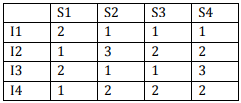# Computer Science Engineering - Online Test

Q1. What is the number of swaps required to sort n elements using selection sort, in the worst case?
Explaination / Solution:
No Explaination.

Q2. Which of the following statement (s) is/are correct regarding Bellman-Ford shortest path algorithm? P. Always find a negative weighted cycle, if one exists. Q. Finds whether any negative weighted cycle is reachable from the source.
Explaination / Solution:
No Explaination.

Q3. Which one of the following languages over the alphabet {0, 1} is described by the regular expression: (0 + 1)* 0 (0 + 1)* 0(0 + 1)*?
Explaination / Solution:
No Explaination.

Q4.
Consider the program below:
#include<stdio.h>
int fun (int n, int *fp)
{
int t, f;
if ( n<= 1)
{
*fp = 1;
return 1;
}
t = fun(n - 1, fp);
f = t+ * fp;
*fp = t;
return f;
}
int main( )
{
int x = 15;
printf (“%d\n”, fun (5, &x));
return 0;
}
The value printed is
Explaination / Solution:
No Explaination.

Q5. One of the purposes of using intermediate code in compilers is to
Explaination / Solution:

Intermediate code is machine independent code which makes it easy to retarget the compiler to generate code for newer and different processors.

Q6. Consider a 4 stage pipeline processor. The number of cycle needed by the four instructions I1,I2,I3,I4 in stages S1, S2, S3, S4 is shown below:What is the number of cycles needed to execute the following loop? for (i=1 to 2) {I1; I2; I3; I4 ;}
Explaination / Solution:
No Explaination.

Q7. The minterm expansion of fExplaination / Solution:
No Explaination.

Q8. A layer-4 firewall (a device that can look at all protocol headers up to the transport layer) CANNOT
Explaination / Solution:

Since it is a layer 4 firewall it cannot block application layer protocol like HTTP.

Q9. The essential content(s) in each entry of a page table is/are
Explaination / Solution:
No Explaination.

Q10. Which one of the following is FALSE?
Explaination / Solution:
No Explaination.